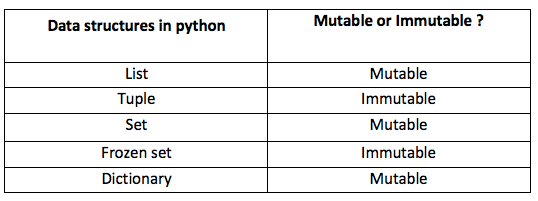Main Features:

• Python works on different platforms (Windows, Mac, Linux, Raspberry Pi, etc).
• Python has a simple syntax similar to the English language.
• Python has syntax that allows developers to write programs with fewer lines than some other programming languages.
• Python runs on an interpreter system, meaning that code can be executed as soon as it is written. This means that prototyping can be very quick.
• Python can be treated in a procedural way, an object-oriented way or a functional way.
• Standards for style: PEP 8

Main Data types:

• Integers
• Floats
• String
• Lists
• Set
• Arrays
• Dictionaries
``````var_int = 1
var_float = 1.1
var_string = "Hello, I am a String"
var_list = [1, 2, "a", 4, 2]
var_array = np.array([1, 2, "a"]) # Some operations are different w.r.t to lists
var_set = set(var_list)
var_dict = {"a" : 10, "b" : 20}
``````

All these structures are mainly divided into two categories.

Mutable Vs. Immutable

Mutable is a fancy way of saying that the internal state of the object is changed/mutated. So, the simplest definition is: An object whose internal state can be changed is mutable. On the other hand, immutable doesn’t allow any change in the object once it has been created.## Jupyter Notebooks and Colab Environment

Notebook documents (or “notebooks”) are documents produced by the Jupyter Notebook App, which contain both computer code (e.g. python) and rich text elements (paragraph, equations, figures, links, etc…). Notebook documents are both human-readable documents containing the analysis description and the results (figures, tables, etc..) as well as executable documents which can be run to perform data analysis.Google Colaboratory, or “Colab” for short, is a product from Google Research. Colab allows anybody to write and execute arbitrary python code through the browser, and is especially well suited to machine learning, data analysis and education. More technically, Colab is a hosted Jupyter notebook service that requires no setup to use, while providing free access to computing resources including GPUs. Link to colab example click here

``````# Variable Definition
a = 10
a
``````
``````print('    /|')
print("   / |")
print("  /  |")
print(" /   |")
print("/____|")
``````
``````# Ask the user for an input
n = input()
print("Hello", n, ", Nice to meet you!")
``````
``````What's your name?
Edoardo
Hello Edoardo , Nice to meet you!
``````
``````# IF Statement
a = 222
b = 200
if b > a:
print("b is greater than a")
elif a > b:
print("a is greater than b")
else:
print("a == b")
``````
``````# For loop
fruits = ["apple", "banana", "cherry"]
for x in fruits:
print(x)

# Or

fruits = ["apple", "banana", "cherry"]
for x in range(len(fruits)):
print(fruits[x])

``````
``````# It is possible to define functions... and even classes

def sum_values(a, b):
sum = a + b
return sum

sum_values(3,4)

``````
``````#  # Executions might be time consuming sometimes...just interrupt them

# import time
# print("Sleeping")
# time.sleep(30) # sleep for a while; interrupt me!
# print("Done Sleeping")
``````
``````# Drive can be mounted as well
drive.mount('/content/drive')

# With free usage, some limits are inevitable:

# Google Colab has a 'maximum lifetime' limit of running notebooks that is 12 hours with the browser open, and the 'Idle' notebook instance is interrupted after 90 minutes.
# In addition, you can have a maximum of 2 notebooks running simultaneously.
``````

## List comprehension

List comprehension offers a shorter syntax when you want to create a new list based on the values of an existing list.

``````fruits = ["apple", "banana", "cherry", "kiwi", "mango"]
newlist = []

for x in fruits:
if "a" in x:
newlist.append(x)

print(newlist)
``````
``````# List comprehensions

fruits = ["apple", "banana", "cherry", "kiwi", "mango"]

newlist = [x for x in fruits if "a" in x]

print(newlist)

# newlist = [expression for item in iterable if condition == True]
``````

## Exercise

a) For example, let’s say we need to create a list of integers which specify the length of each word in a certain sentence, but only if the word is not the word for”.

``````sentence = "Big Data Management for Executive"
words = sentence.split()
word_lengths = []
for word in words:
if word != "the":
word_lengths.append(len(word))
print(words)
print(word_lengths)
``````

Using a list comprehension, we could simplify this process..

b) Using a list comprehension, create a new list called “newlist” out of the list “numbers”, which contains only the positive numbers from the list, as integers (int function).

``````numbers = [34.6, -203.4, 44.9, 68.3, -12.2, 44.6, 12.7]
newlist = []
print(newlist)
``````
``````# sentence = "Big Data Management for Executive"
# words = sentence.split()
# word_lengths = []
# for word in words:
#       if word != "for":
#           word_lengths.append(len(word))
# print(words)
# print(word_lengths)

# numbers = [34.6, -203.4, 44.9, 68.3, -12.2, 44.6, 12.7]
# newlist = [int(x) for x in numbers if x > 0]
# print(newlist)
``````Tags: